## 10+ Equations of Reality

/ The world based on numbers and relations /

μάθημα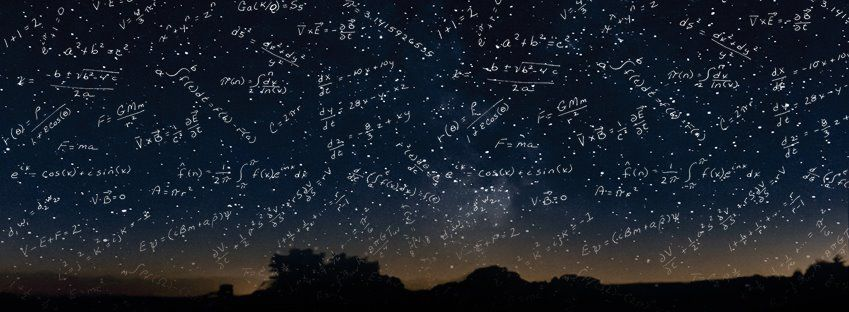11:15, restate my assumptions: 1. Mathematics is the language of nature; 2. Everything around us can be represented and understood through numbers; 3. If you graph these numbers, patterns emerge. Therefore: There are patterns everywhere in nature.

– Max Cohen

Mathematics (from the Greek word: mathema) means knowledge, study, learning and includes the study of such topics as quantity, structure, space and change. It seek and use patterns to formulate new conjectures; they resolve the truth or falsity of conjectures by mathematical proof. When mathematical structures are good models of real phenomena, then mathematical reasoning can provide insight or predictions about nature. Through the use of abstraction and logic, mathematics developed from counting, calculation, measurement, and the systematic study of the shapes and motions of physical objects. Practical mathematics has been a human activity from as far back as written records exist.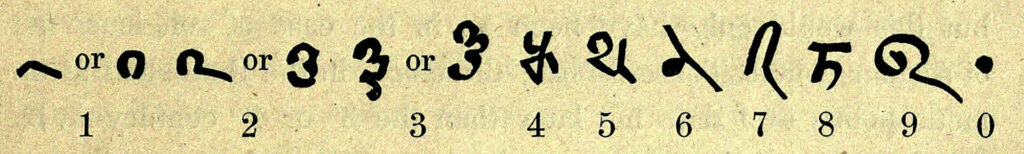The research required to solve mathematical problems can take years or even centuries of sustained inquiry. The brightest minds in history have used mathematics to lay the foundation for now we measure and understand our universe.

Time and time again, we have proved that it only takes one simple formula to alter the course of humanity:

.

#### Isaac Newton’s Law of Universal Gravitation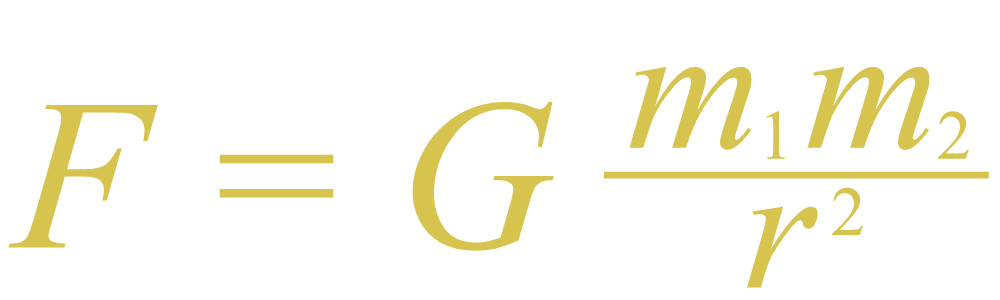Newton’s law explains why the planets move the way they do and how gravity works, both on Earth and throughout the universe. First published in the ”Principia” in July 1687, the Law of Universal Gravitation was the defacto reference equation for nearly 200 years until Einstein’s Theory of General Relativity replaced it.

#### Albert Einstein’s Theory of Relativity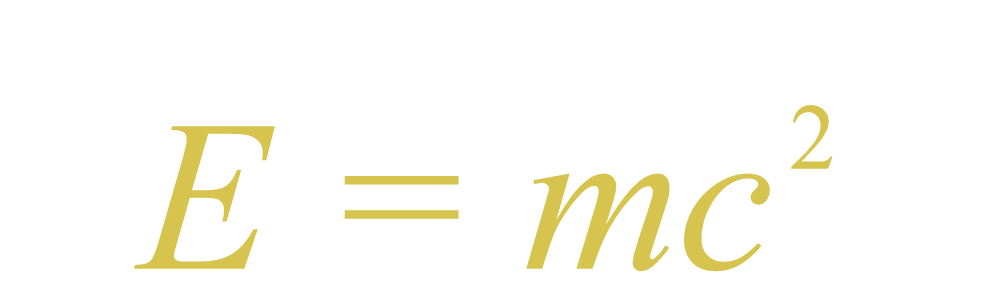Einstein’s most famous undertaking is the generally accepted theory on the relationship between space and time. First proposed in 1905, the Theory of Relativity has both radically altered the course of physics and deepened our knowledge of the universe’s past, present and future.

.

.

#### The Pythagorean Theorem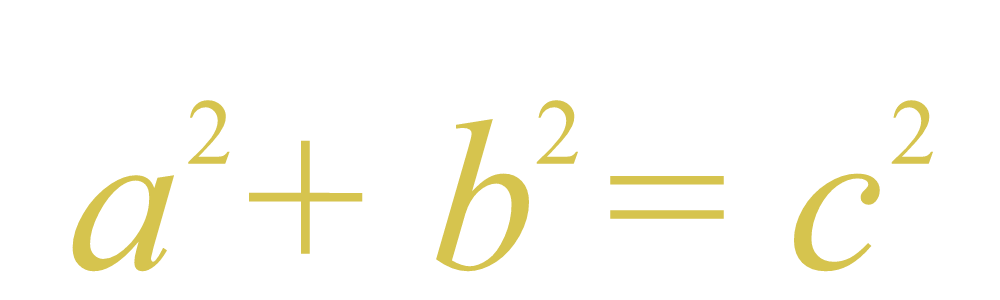This ancient – first recorded circa 570-495 BC – is a fundamental principle in Euclidean Geometry and the basis for the definition of distance between two points. Pythagora’s theorem also describes the relationship between the sides of a right triangle on a flat plane.

#### Maxwell’s Equations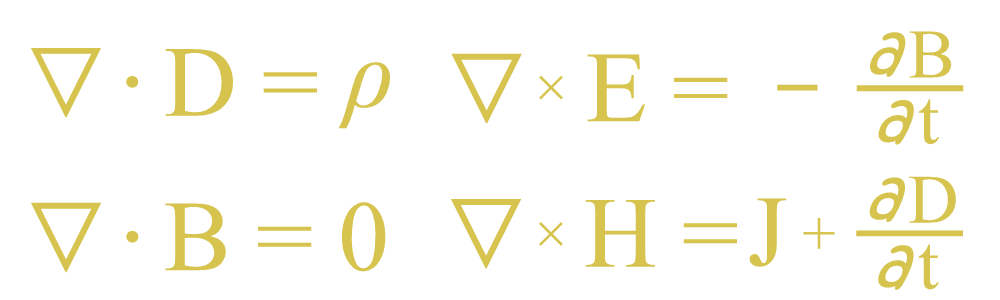James Clerk Maxwell’s set of equations describe how electric and magnetic fields are generated and altered, both by each other and by charges and currents. First published between 1861 and 1862, they are to classical electromagnetism what Newton’s laws of motion and universal gravitation are to classical mechanics.

.

.

#### The Second Law of ThermodynamicsRudolf Clausius’ law states that energy always flows from higher concentration to lower concentrations. It also states that whenever energy changes or moves, it becomes less useful. Formulated in 1865, it has led to the development of technologies like internal combustion engines, cryogenics and electricity generation.

#### Logarithms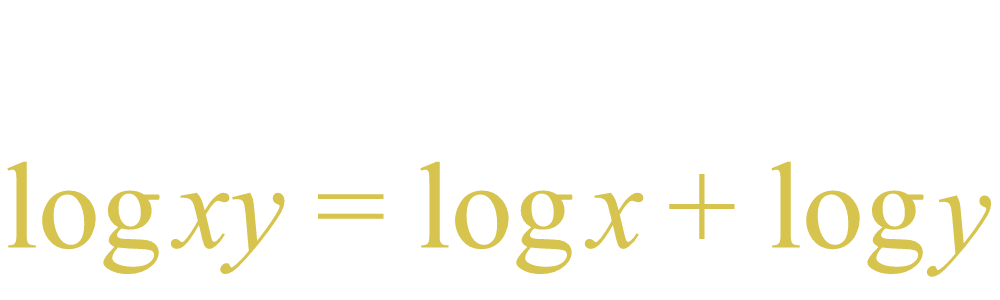Logarithms were introduced by John Napier in the early 17th century as a way to simplify calculations. They answer the question, ”How many of X number do we multipy to get Y number?” Logarithms were adopted by early navigators, scientists and engineers. Today, scientific calculators and digital computers do the work for us.

.

.

#### Calculus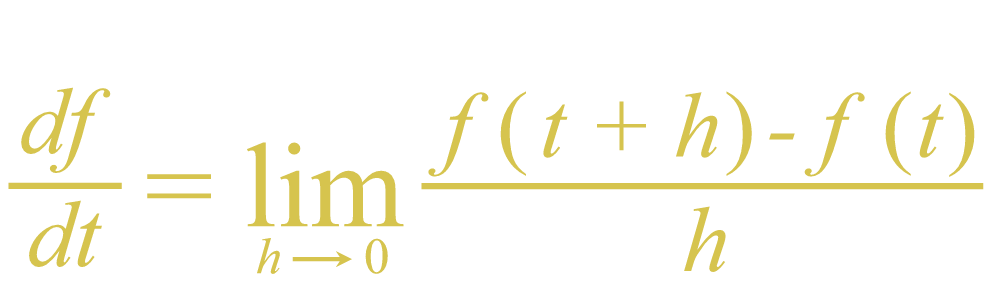The calculation shown is the definition of the derivative in differential calculus, one of calculus’ two major branches. The derivative measures the rate at which a quantity is changing — if you are walking 2 km an hour, then you will change your position by 2 km every hour. In the 1600s, Newton used calculus to develop his laws of motion and gravitation.

#### Schrödinger Equation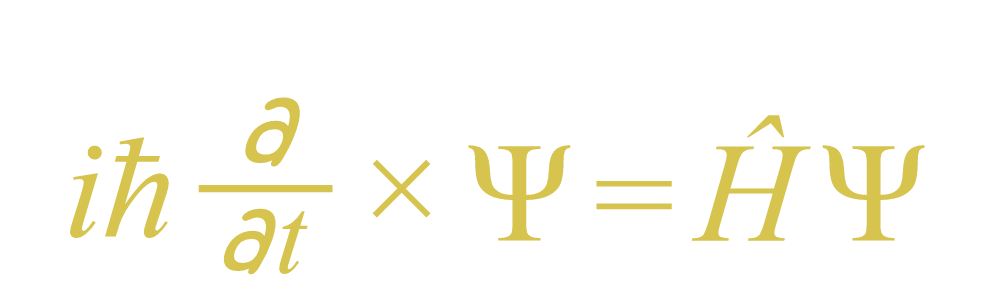This equation describes how the quantum state of a quantum system changes with time. Developed by Austrian physicist Erwin Schrödinger in 1926, it governs the behavior of atoms and subatomic particles in quantum mechanics. Schrodinger’s Equation paved the way for nuclear power, microchips, electron microscopes, and quantum computing.

.

.

#### Information Theory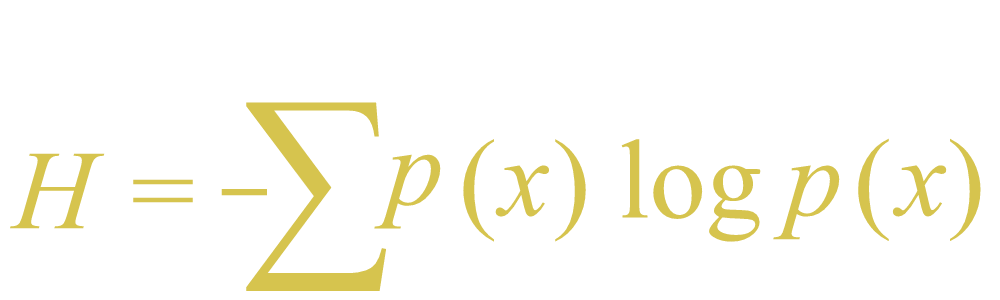Information theory is a branch of mathematics that studies the coding of information in the form of sequences of symbols, and the speed at which that information can be transmitted. Applications of topics within information theory include data compression and channel coding. Research in the field was also instrumental in the development of the Internet and mobile phones.

#### Chaos Theory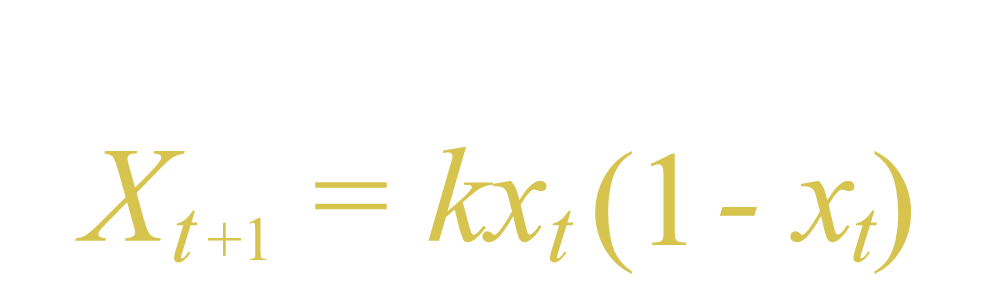Chaos Theory is a branch of mathematics that studies complex systems whose behavior is extremely sensitive to slight changes in conditions. In essence, it shows how small alterations can lead to consequences of much greater scale. Chaos Theory has applications just about everywhere — meteorology, sociology, physics, computer science, engineering, economics, biology, and philosophy.

.

#### Dirac Equation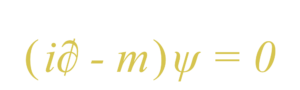In particle physics, the Dirac equation is a relativistic wave equation derived by British physicist Paul Dirac in 1928. The equation also implied the existence of a new form of matter, antimatter, previously unsuspected and unobserved and which was experimentally confirmed several years later. It also provided a theoretical justification for the introduction of several component wave functions in Pauli’s phenomenological theory of spin.

OTHER CONCEPTS

Pratite diskusiju/Subscribe
Obaveštavaj/Notify